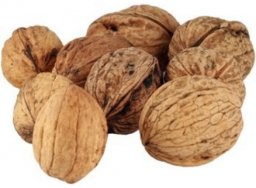Two brothers

The two brothers have a total of 48 nuts. Two thirds of the nuts of the first correspond to 2/5 of the number of nuts of the second. How many nuts does everyone have?

a =  18
b =  30

Step-by-step explanation:Did you find an error or inaccuracy? Feel free to write us. Thank you!Tips to related online calculators
Need help to calculate sum, simplify or multiply fractions? Try our fraction calculator.
Do you have a system of equations and looking for calculator system of linear equations?